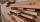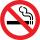# Mass + third power - math problems

#### Number of problems found: 11

• Density 2What is the density of a piece of driftwood that has the mass of 25 grams and a volume is 30 cm to the 3rd power. And how do I figure it out?
• CubeThe cube weighs 11 kg. How weight is a cube of the same material if its dimensions are 3-times smaller?
• Hollow sphereCalculate the weight of a hollow stříbrné sphere (density 10.5 g/cm3) if the vnitřní diameter is 15 cm and wall thickness is 1 mm.
• Granite cubeWhat is the weight in kg granite cube with an edge of 0.5 m if 1dm3 of granite weight 2600 g?
• SphereThe surface of the sphere is 12100 cm2, and the weight is 136 kg. What is its density?
• The half lifeThe half-life of a radioactive isotope is the time it takes for a quantity of the isotope to be reduced to half its initial mass. Starting with 145 grams of a radioactive isotope, how much will be left after 3 half-lives?
• Wall thicknessThe hollow metal ball has an outside diameter of 40 cm. Determine the wall thickness if the weight is 25 kg and the metal density is 8.45 g/cm3.
• Iron sphereIron sphere has weight 100 kg and density ρ = 7600 kg/m3. Calculate the volume, surface and diameter of the sphere.
• No smokeTobacco company NO-SMOKE adorned its stand at the cigarette-type trade fair with the cigarette-shaped. The dimensions of which were 20 times the size of a regular cigarette. The regular cigarette contains 0.8 mg of nicotine. How much nicotine would a gian
• Hollow sphereThe steel hollow sphere floats on the water plunged into half its volume. Determine the outer radius of the sphere and wall thickness, if you know that the weight of the sphere is 0.5 kg and the density of steel is 7850 kg/m3
• Tower modelTower height is 300 meters, weight 8000 tons. How high is the model of the tower weight 1 kg? (State the result in the centimeters). The model is made from exactly the same material as the original no numbers need to be rounded. The result is a three-digi

We apologize, but in this category are not a lot of examples.
Do you have an interesting mathematical word problem that you can't solve it? Submit a math problem, and we can try to solve it.

We will send a solution to your e-mail address. Solved examples are also published here. Please enter the e-mail correctly and check whether you don't have a full mailbox.

Please do not submit problems from current active competitions such as Mathematical Olympiad, correspondence seminars etc...

Mass - math word problems. Third power - math word problems.